yǐn yòng

quote

• ## 引用（C++学习笔记 15）

千次阅读 多人点赞 2018-11-05 18:27:21
一、引用的作用 建立引用的作用是为变量另起一个名字，变量的引用通常被认为是变量的别名。 对变量声明一个引用，并不另外开辟内存单元，变量 i 和引用 j 占用内存的同一位置，当 i 变化时，j 也随之变化， 反之...
一、引用的作用
建立引用的作用是为变量另起一个名字，变量的引用通常被认为是变量的别名。
对变量声明一个引用，并不另外开辟内存单元，变量 i 和引用 j 占用内存的同一位置，当 i 变化时，j 也随之变化， 反之亦然。
二、引用的格式
类型 &引用名=已定义的变量名；
注意：（1）在声明引用时，必须立即对它进行初始化，不能声明完成后再赋值。
（2）为引用提供的初始值可以是变量或另一个引用。
（3）指针是通过地址间接访问某个变量，而引用是通过别名直接访问某个变量，使用引用可以简化程序。（因为不用书写“*”）。
（4）引用在初始化后不能再重新声明为另一个变量的引用（别名）。
（5）可以将引用的地址赋给一个指针，此时指针指向原来的变量。
（6）引用仅在声明时带有引用运算符“&”，以后像普通变量一样使用，不能再带“&”，其它场合使用的“&”都是地址操作符。
例如： 声明一个 j 作为 i 的别名。
int i=5;
int &j=i;  //声明j是一个整型变量的引用，用整形变量i对它进行初始化

例1： 变量和引用的关系。
#include<iostream>
using namespace std;
int main(){
int i;
int &j=i;
i=30;
cout<<"i="<<i<<" j="<<j<<endl;
j=80;
cout<<"i="<<i<<" j="<<j<<endl;
}

执行结果：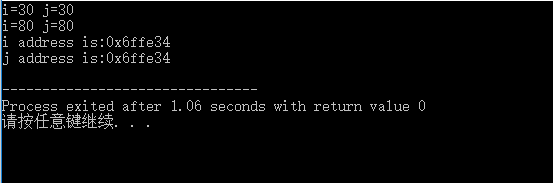三、引用作为函数参数
例2： 指针变量作为函数参数的例子。
#include<iostream>
using namespace std;
void swap(int *m,int *n){
int temp;
temp=*m;
*m=*n;
*n=temp;
}
int main(){
int a=5,b=10;
cout<<"a="<<a<<" b="<<b<<endl;
swap(&a,&b);
cout<<"a="<<a<<" b="<<b<<endl;
return 0;
}

执行结果如下图：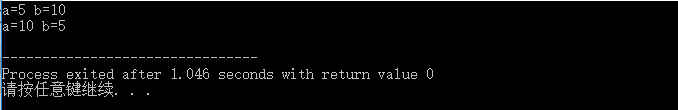例3： 引用作为函数参数的例子。
#include<iostream>
using namespace std;
void swap(int &m,int &n){
int temp;
temp=m;
m=n;
n=temp;
}
int main(){
int a=5,b=10;
cout<<"a="<<a<<" b="<<b<<endl;
swap(a,b);
cout<<"a="<<a<<" b="<<b<<endl;
return 0;
}

执行结果和例2一致。
在该例子中对m和n的访问实际上就是对a和b的访问。
四、使用引用返回函数值
例3： 使用引用返回函数值。
#include<iostream>
using namespace std;
int a[]={1,3,5,7,9};
int &index(int);  //声明函数，返回一个整数类型的引用
int main(){
index(2)=25; //函数为引用时，才可以将函数调用放在"="左边
cout<<index(2);
return 0;
}
int &index(int i){
return a[i];
}

五、引用举例
例4： 应用引用的综合例子。
#include<iostream>
using namespace std;
int &max(int &num1,int &num2);
int &min(int &num1,int &num2);
main(){
int num1,num2;
cout<<"请输入第1个数:";
cin>>num1;
cout<<"请输入第2个数:";
cin>>num2;
max(num1,num2)=0;
cout<<"\n找出最大数，然后把最大数赋值为0后，两个数分别为："<<endl;
cout<<num1<<"和"<<num2<<endl;
cout<<"\n现在，请再输入两个数："<<endl;
cout<<"请输入第1个数:";
cin>>num1;
cout<<"请输入第2个数:";
cin>>num2;
min(num1,num2)=0;
cout<<"\n找出最小数，然后把最小数赋值为0后，两个数分别为："<<endl;
cout<<num1<<"和"<<num2<<endl;
return 0;
}
int &max(int &num1,int &num2){
return(num1>num2)?num1:num2;
}
int &min(int &num1,int &num2){
return(num1<num2)?num1:num2;
}

执行结果如下图所示：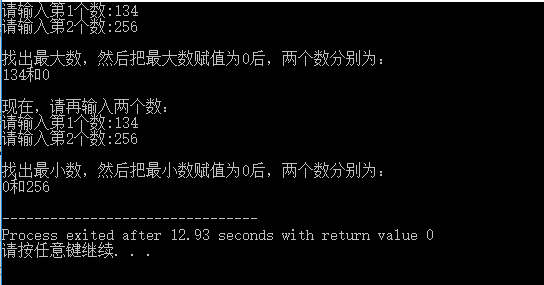展开全文• C/C++ *和& 详解 C/C++中 * 的用法 1>最简单的乘法： a*b; 2>可以注释： /**/ 3>指针：（最重要） 指针是指向变量的地址 简单的例子分析： int main() { int a = 3...


C/C++ *和& 详解

C/C++中 *  的用法
1>最简单的乘法：
a*b;
2>可以注释：
/**/
3>指针：（最重要）
指针是指向变量的地址
简单的例子分析：
int main()
{
int a = 3;
int *b = &a;
cout << "a：" << a << endl;
cout << "b：" << b << endl;
*b = 10;
cout << "&a：" << &a << endl;
cout << "b：" << b << endl
结果：
a：3
b：00EFFE28
&a：00EFFE28
b：00EFFE28
a：10
分析：
b是a的指针，指向a的地址。（也就是a与b相连，只要修改*b的值，a的值也跟着改动）
C/C++中 & 的用法
1>位运算符
a&b
2>逻辑与 &&
if((a==0)&&(b==0)){}
1
3>引用  &（最重要）
引用可说把a变量换了一个名字为b即：
&b=a
1
简单的例子分析：
//引用
int main()
{
int a = 3;
int &b = a;
int c = a;
cout << "a：" << a << endl;
cout << "b：" << b << endl;
cout << "c：" << c << endl;
b = 10;
cout << "a：" << a << endl;
cout << "b：" << b << endl;
cout << "c：" << c << endl;
cout << "&a：" << &a << endl;
cout << "&b：" << &b << endl;
cout << "&c：" << &c << endl;
system("pause");
}
结果：
a：3
b：3
c：3
a：10
b：10
c：3
&a：0019FD74
&b：0019FD74
&c：0019FD5C
分析：
& 引用  ：比如说，一个人有多少外号，但都是指这个人，引用也是这样。如果b的值改动了，也就代表了a的值改动了。
（核心）函数的参数
1>函数传入普通参数
//函数传入普通参数
void fun(int a,int b)
{
int c = 0;
c = a;
a = b;
b = c;
}
int main()
{
int a = 1;
int b = 2;
cout << a << "," << b << endl;
fun(a, b);//a,b交换
cout << a << "," << b << endl;
system("pause");
return 0;
}
结果：
1,2
1,2
分析:
函数传入的是形参，不会改变main()中a,b的地址，也就是不会改变a,b的值。
2>函数传入指针参数
//函数传入指针参数
void fun(int *a, int *b)
{
int c = 0;
c = *a;
*a = *b;
*b = c;
}
int main()
{
int a = 1;
int b = 2;
cout << a << "," << b << endl;
fun(&a, &b);//a,b交换
cout << a << "," << b << endl;
system("pause");
return 0;
}
结果：
1,2
2,1
分析:
函数的参数传入的是指针，即地址。函数中a,b的交换是地址的交换。最后main()中的a,b的值也就改变了。
3>引用传入函数参数(简单–好操作)
//引用传入函数参数
void fun(int &a, int &b)
{
int c = 0;
c = a;
a = b;
b = c;
}
int main()
{
int a = 1;
int b = 2;
cout << a << "," << b << endl;
fun(a, b);//a,b交换
cout << a << "," << b << endl;
system("pause");
return 0;
}
结果：
1,2
2,1
分析:
本质上是把main()中的a,b变量 换了个名字，即：函数中的a,b，函数中的a,b地址与main()中的地址是一样的。如果函数中的a,b值改变的，那么main()中的a,b的值也跟着改变。
这一篇博客总结了初接触c/c++ 的指针，地址，引用  最为疑惑和困难的地方。同时，也是最重要的知识。也希望大家有什么不懂了及时留言，作者看到了也会及时回复的，如有什么错误的，也欢迎指正。


展开全文c++
•   引用不是新定义一个变量，而是给已存在变量取了一个别名，编译器不会为引用变量开辟内存空间，它和它引用的变量共用同一块内存空间。 #include&lt;iostream&gt; using namespace std; int main() { ...
** 引用的定义：**
引用不是新定义一个变量，而是给已存在变量取了一个别名，编译器不会为引用变量开辟内存空间，它和它引用的变量共用同一块内存空间。
#include<iostream>
using namespace std;

int main()
{
int a = 10;
int& ra = a;
a = 20; //a, ra 都改为20，a，ra的地址一样
return 0;
}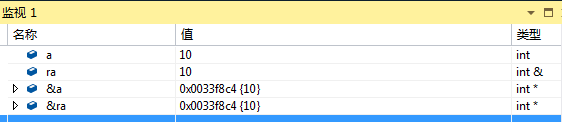引用的特征

引用在定义的时候必须初始化
一个变量可以有多个引用
引用一旦引用某个实体，再不能引用其他实体

常引用
#include<iostream>
using namespace std;

int main()
{
const int a = 10;
const int& ra = a;
const int& c = 10;//引用一个常数
int b = 10;
const double& rb = b;//类型的引用，b先转换成一个常量，rb是常量的别名
b = 20;//rb不改变
return 0;
}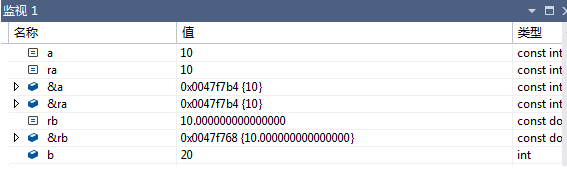引用的使用

作参数
不需要另外开闭空间，实参只是形参的别名

void Swap(int& left, int& right)
{
int temp = left;
left = right;
right = temp;
}


作返回值
函数的栈帧调用结束之后，变量必须依然存在，否则不能返回引用

int& TestRefReturn(int& a)
{
a += 10;
return a;
}

传值和传引用的比较
在传值作形参的时候，形参是实参的一份临时拷贝，需要开闭空间，而引用只是一个别名，不需要开闭空间，效率高。
在传值作返回值的时候，需要开闭空间，函数的栈帧结束之后，栈上的空间已经归还系统，因此返回值是另外开辟了空间保存返回值，而用引用做返回值的条件是，函数调用结束之后，改变量或者对象必须依然存在，不需要开辟空间，效率高。
指针和引用的区别
a.在语法上指针开辟了空间，保存了变量的地址；引用没有开辟空间，只是起了一个别名
b.在底层实现上，引用是按照指针实现的，两者的汇编代码一致

引用在定义时必须初始化，指针没有要求
引用在初始化时引用一个实体后，就不能再引用其他实体，而指针可以在任何时候指向任何一个同类型实体
没有NULL引用，但有NULL指针
在sizeof中含义不同：引用结果为引用类型的大小，但指针始终是地址空间所占字节个数(32位平台下占4个字节)
引用自加即引用的实体增加1，指针自加即指针向后偏移一个类型的大小
有多级指针，但是没有多级引用
访问实体方式不同，指针需要显式解引用，引用编译器自己处理
引用比指针使用起来相对更安全


展开全文• 一、引用的概念 引用不是定义一个变量，而是给一个已有成员起一个别名 类型 &amp;引用变量名 = 以定义的变量名 int a = 10; int &amp;b = a; 引用 的特点 1.一个变量可以有多个成员 2.引用必须初始化...
一、引用的概念

引用不是定义一个变量，而是给一个已有成员起一个别名

类型 &引用变量名 = 以定义的变量名

int a = 10;
int &b = a;

引用 的特点

1.一个变量可以有多个成员

2.引用必须初始化

3.引用只能在初始化的时候引用一次，不能改变在引用其他的变量

二、const 引用

1.const引用 可以为  非const变量  的别名

非const变量相当于可以对他进行读、写操作

而const引用，只能对他进行读，不能进行写操作

权限     读写->只读（权限可以缩小）

        int a = 4;
const int& b = a;
cout << a << endl;  //4
cout << b << endl;  //4

变量的值被改变时，引用的值同时也会改变，但是不能直接改变引用的值

        a = 5;
cout << a << endl; //5
cout << b << endl; //5
//b = 6;    //不能对引用进行赋值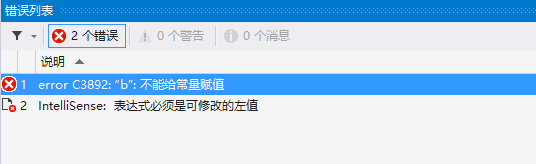2. const变量只能用const引用，不能使用非const引用

const变量相当于只有读的权限，没有写的权限

非const引用既有读，又有写的权限

权限 ：读->读写（不可以）

权限只能放大，不能缩小

        const int c = 1;
const int& d = c;
//int& d1 = c;
const int & d2 = 5;//常量具有常性，只有常引用可以引用常量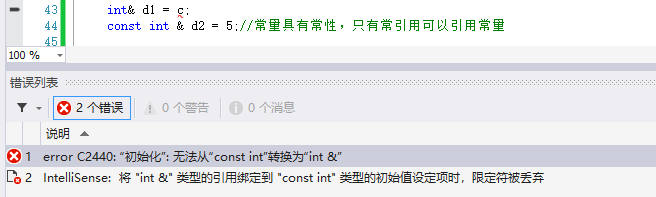3.不同类型变量引用要用const

        double a = 1.1;
//int &b = a;
const int& b1 = a;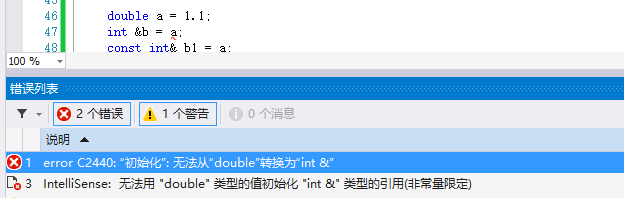a是double类型的，它要赋值给int类型会发生隐式类型转换

用a赋值给b时，会生成一个临时变量，即b引用的时这个带有常性的临时变量，所以要用const

三、引用做参数

1.值传递

void Swap1(int left, int right)
{
int temp = left;
left = right;
right = temp;
}

int main()
{
int a = 10;
int b = 20;
cout << "a:" << a << endl;
cout << "b:" << b << endl;
Swap1(a, b);

cout << "a:" << a << endl;
cout << "b:" << b << endl;
system("pause");
return 0;
}

值传递不能改变a，b的值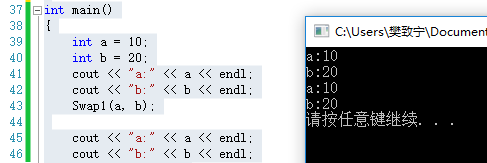2.引用传递

void Swap2(int& left, int& right)
{
int temp = left;
left = right;
right = temp;
}

int main()
{
int a = 10;
int b = 20;
cout << "a:" << a << endl;
cout << "b:" << b << endl;
Swap2(a, b);

cout << "a:" << a << endl;
cout << "b:" << b << endl;
system("pause");
return 0;
}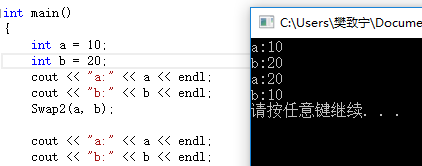3.指针传递

void Swap3(int* left, int* right)
{
int temp = *left;
*left = *right;
*right = temp;
}

int main()
{
int a = 10;
int b = 20;
cout << "a:" << a << endl;
cout << "b:" << b << endl;
Swap3(&a, &b);

cout << "a:" << a << endl;
cout << "b:" << b << endl;
system("pause");
return 0;
}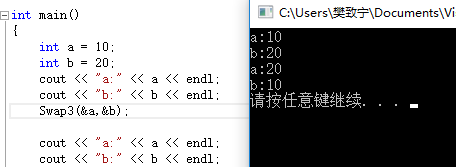引用传递和值传递

引用传递效率更高，值传递会有对象变量的拷贝，生成局部变量；

而引用是参数的一个别名，本身不占内存，所以开销小。

四、引用做返回值

1、正常的值返回

int Add(int a, int b)
{
int c = a + b;
return c;
}
int main()
{
int a = 10;
int b = 20;
const int& ret = Add(a, b);
cout << ret;
system("pause");
return 0;
}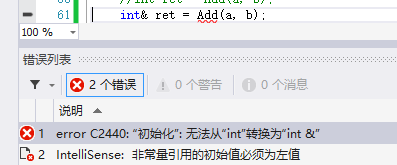2.引用做返回值

int& Add(int a, int b)
{
int c = a + b;
return c;
}
int main()
{
int a = 10;
int b = 20;
cout << ret;
system("pause");
return 0;
}

注：尽量不要返回临时变量的引用，如果不是临时变量，则可以用引用返回

因为临时变量出了作用域以后就会销毁，临时变量的引用相当于它的别名，变量销毁了以后，引用就会指向不确定的内存
展开全文• ## 什么是引用？

千次阅读 2019-06-21 14:21:00
引用：为对象起立另一个名字，引用类型引用另一种类型。 引用并非对象，相反的，它只是为一个已存在的对象所起的另外一个名字。如图1-1。 图 1-1 引用必须进行初始化，定义了一个引用之后，对其进行的所有操作...
• ## Java的四种引用方式

千次阅读 多人点赞 2019-03-16 09:34:23
java内存管理分为内存分配和内存回收，都不需要程序员负责，垃圾回收的机制主要是看对象是否有引用指向该对象。 java对象的引用包括： 强引用引用引用 虚引用 Java中提供这四种引用类型主要有两个目的： ...
• ## 引用

2019-07-18 17:15:21
引用不是新定义一个变量，而是给已存在变量取了一个别名，编译器不会为引用变量开辟内存空间，它和它引用的变量共用同一块内存空间。 类型& 引用变量名(对象名) = 引用实体； void TestRef() { int a = 10;...
• 引用的效果就如本文档中代码和样式示例。在每行行首加‘&gt;’。 &gt;这是一则引用 &gt;每行行尾没有双空格 &gt;所以会显示为一行引用 显示为 这是一则引用 每行行尾没有双空格 ...
• ## C/C++引用

千次阅读 2019-04-24 10:57:06
引用符号：&，出现在类型之后 Struct Object { int value; }; int main { Object a={1}; Object& r=a; return 0; } 1、创建一个类型为Object的对象a，变量r是对象a的一个引用（总共只有一个对象，r...
• \usepackage[super,square]{natbib}%%%参考文献引用格式调整 \begin{document} 正文\cite{huang},正文\citep{liuhaiyang} \begin{thebibliography}{99} \bibitem{huang} PDF：黄新刚的\LaTeX Notes：生动有趣 \...参考文献
• 什么是引用？ int a; int &b=a; 上边就是变量的引用 符号&不是去地址的含义了，去地址是在指针时的含义，这里表示引用。（这个引用不是动词，而是名词） 引用的定义：对一个数据可以建立一个“引用”，它的作用是为...
• ## 走进java_符号引用与直接引用

万次阅读 多人点赞 2017-05-28 14:55:27
Java类从加载到虚拟机内存中开始，到... 而在解析阶段会有一个步将常量池当中二进制数据当中的符号引用转化为直接引用的过程。 符号引用 ：符号引用以一组符号来描述所引用的目标。符号引用可以是任何形式的字面量，
• Java中只有传值调用（值传递），没有传址调用（址传递或者引用传递）。所以在java方法中改变参数的值是不会改变原变量的值的，但为什么改变引用变量的属性值却可以呢？请看下面的解答。 java中的数据类型 Java中...java值传递
• 作为一个有着 8 年 Java 编程经验的 IT 老兵，说起来很惭愧，我被 Java 当中的四五个名词一直困扰着：**对象、引用、堆、栈、堆栈**（栈可同堆栈，因此是四个名词，也是五个名词）。每次我看到这几个名词，都隐隐...java 对象
• ## C++11 右值引用&&

万次阅读 多人点赞 2017-11-13 14:43:33
右值引用 (Rvalue Referene) 是 C++ 新标准 (C++11, 11 代表 2011 年 ) 中引入的新特性 , 它实现了转移语义 (Move Sementics) 和精确传递 (Perfect Forwarding)。它的主要目的有两个方面： 1. 消除两个对象交互时...c++11
• //发现是引用，转换为int* const ref = &a; void func(int& ref) { ref = 100; // ref是引用，转换为*ref = 100 } int main() { int a = 10; //自动转换为int* const ref = &a; 指针常量是...c++
• ## C++ 常量引用

万次阅读 2020-07-04 19:11:18
//常量引用 //使用场景:用来修饰形参，防止误操作 //int a = 10; //加上const之后编译器将代码修改int temp = 10; const int & ref = temp; const int & ref = 10;//引用必须引一块合法的内存空间 ...C++
• 引用计数：Java堆中每一个对象都有一个引用计数属性，引用每新增1次计数加1，引用每释放1次计数减1。 在JDK 1.2以前的版本中，若一个对象不被任何变量引用，那么程序就无法再使用这个对象。也就是说，只有对象...Java
• ## C++ 函数的引用传递

万次阅读 2020-07-04 11:44:00
#include<iostream> #include<string> using namespace std; //1、值传递 void mySwap01(int a, int b) { int temp = a; a = b; b = temp; cout << "mySwap01 a=" <...}.c++
• 一、强引用 二、软引用 三、弱引用 四、虚引用 五、总结 在JDK1.2以前的版本中，当一个对象不被任何变量引用，那么程序就无法再使用这个对象。也就是说，只有对象处于可触及状态，程序才能使用它。这就像在商店...java...